# Texas Go Math Grade 2 Lesson 14.4 Answer Key Draw Two-Dimensional Shapes

Refer to our Texas Go Math Grade 2 Answer Key Pdf to score good marks in the exams. Test yourself by practicing the problems from Texas Go Math Grade 2 Lesson 14.4 Answer Key Draw Two-Dimensional Shapes.

## Texas Go Math Grade 2 Lesson 14.4 Answer Key Draw Two-Dimensional Shapes

Explore

Write the number of sides and vertices. Use the word list to name the shape.

hexagon
octagon
pentagon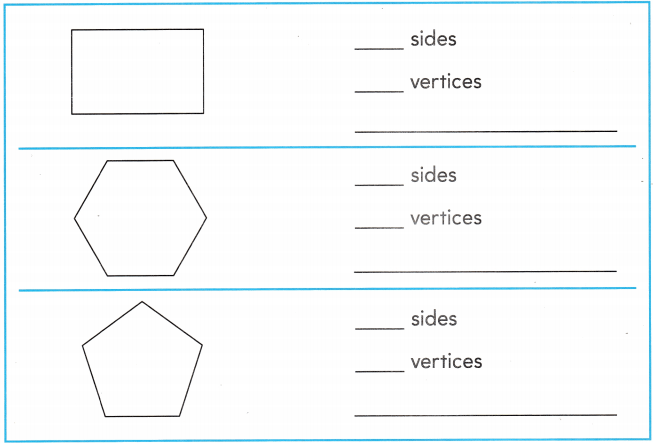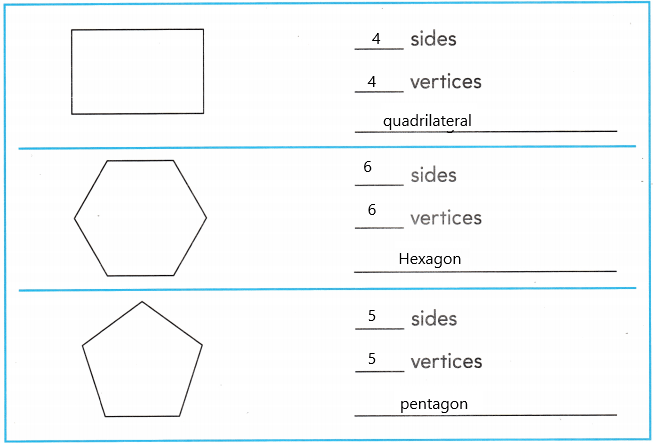Explanation:
A quadrilateral has 4 sides and 4 vertices, a hexagon has 6 sides and 6 vertices and a pentagon has 5 sidesand 5 vertices.

FOR THE TEACHER • Read the following problem. Tracey draws three shapes. What are the shapes that Tracey draws?

Math Talk
Mathematical Processes

Explain how you know the names of the three shapes.

Model and Draw

Here is a shape with 4 vertices. Draw a different shape with 4 vertices.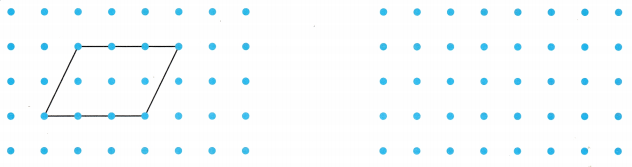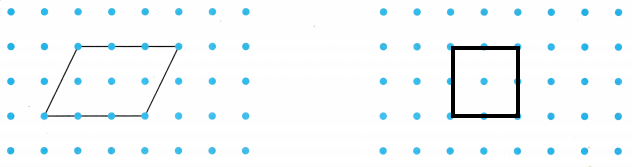Explanation:
I drew a square. A square has 4 sides.

Share and Show

Use a ruler to draw the shape.

Question 1.
a shape with 5 sides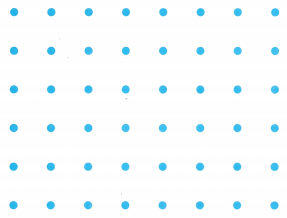Explanation:
A shape with 5 sides is a pentagon. So, i drew a pentagon.

Question 2.
a shape with 3 verticesExplanation:
A shape with 3 sides is a triangle. So, I drew a triangle.

Go Math Grade 2 Answer Key Draw 2 Dimensional Shapes Question 3.
a shape with 8 vertices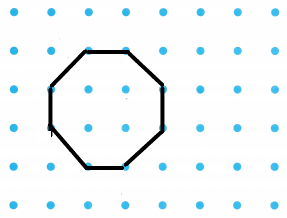Explanation:
A shape with 8 sides is a octagon. So, i drew a octagon.

Question 4.
a shape with 6 sides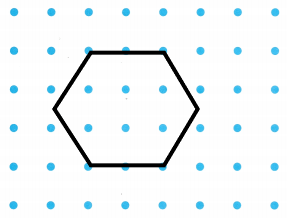Explanation:
A shape with 6 sides is a hexagon. So, i drew a hexagon.

Problem Solving

Use a ruler to draw the shape.

Question 5.
a shape with 5 verticesExplanation:
A shape with 5 vertices is a pentagon. So, i drew a pentagon.

Question 6.
a shape with 7 sides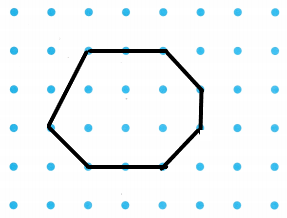Explanation:
I drew a shape with 7 sides.

Go Math 2nd Grade Answer Key Draw 2 Dimensional Shapes Question 7.
H.O.T. Multi-Step Janet drew a pentagon and a hexagon. How many sides did she draw? Draw to prove your answer.
_________ sides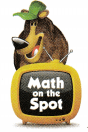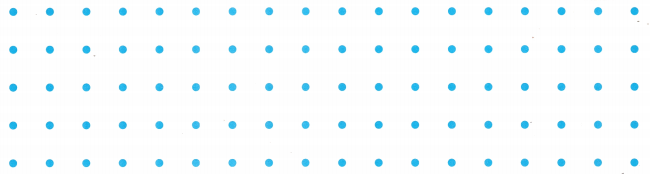Explanation:
Janet drew a pentagon and a hexagon. There are 12 sides in all.

Question 8.
H.O.T. Multi-Step Ben drew 3 shapes that had 14- sides in all. Draw shapes Ben could have drawn.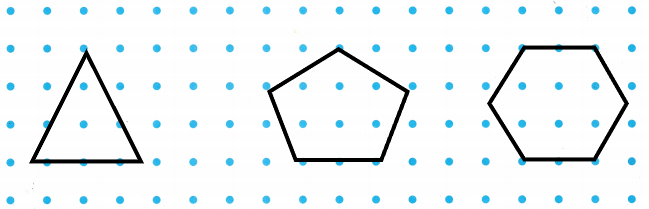Explanation:
Ben drew 3 shapes that had 14- sides in all. Ben could have drawn a triangle, pentagon and a hexagon.

Question 9.
Use Diagrams Alex drew a picture to show the shape of a garden. How many sides does the garden have?(A) 5 sides
(B) 8 sides
(C) 6 sides
(B) 8 sides

Explanation:
Alex drew a picture to show the shape of a garden. It has 8 sides.

Question 10.
Use a ruler. Draw a shape with 8 sides. Then draw a shape with 5 vertices.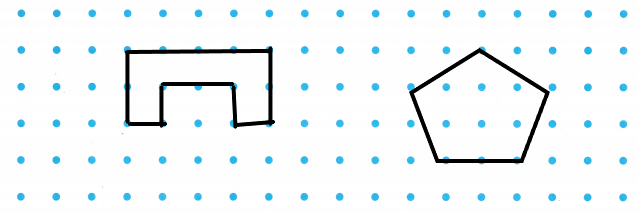Explanation:
I drew a shape with 8 sides and a shape with 5 vertices.

Texas Test Prep Terry draws this shape. How many vertices does the shape have?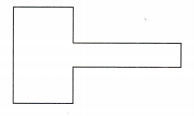(A) 8 vertices
(B) 4 vertices
(C) 6 vertices
(A) 8 vertices

Explanation:
Terry draws this shape. It has 8 vertices.

TAKE HOME ACTIVITY • Have your child explain how he or she solved one of the problems in this lesson.

### Texas Go Math Grade 2 Lesson 14.4 Homework and Practice Answer Key

Use a ruler to draw the shape.

Question 1.
a shape with 6 vertices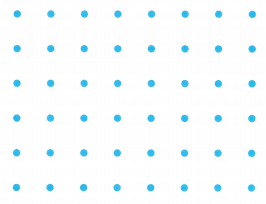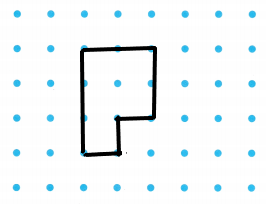Explanation:
I drew a shape with 6 vertices.

Question 2.
a shape with 9 sides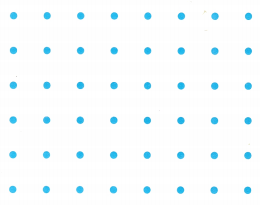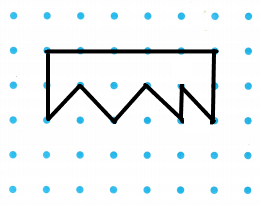Explanation:
I drew a shape with 9 sides.

Problem Solving

Question 3.
Multi-Step Laura drew a hexagon and an octagon. How many sides did she draw? Draw to prove your answer.
____________ sides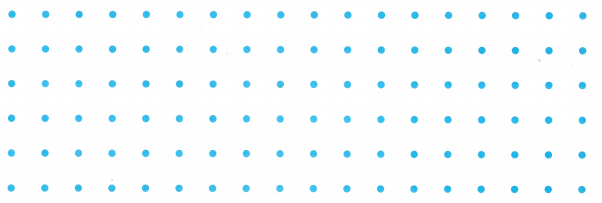Explanation:
Laura drew a hexagon and an octagon. She drew 14 sides.

Lesson Check

Question 4.
Henry draws this shape. How many sides does the shape have?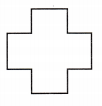(A) 11
(B) 12
(C) 8
(B) 12

Explanation:
Henry draws this shape. It has 12 sides in all.

Question 5.
Melinda draws a picture of her comb. How many vertices does this shape have?(A) 9
(B) 7
(C) 10
(A) 9

Explanation:
Melinda draws a picture of her comb. It has 9 vertices.

Go Math Grade 2 Chapter 14 Lesson 14.4 Answer Key Question 6.
Nate draws his bedroom. How many sides does his bedroom have?(A) 4
(B) 8
(C) 6
(C) 6

Explanation:
Nate draws his bedroom. It has 6 sides.

Question 7.
Keith draws a baseball field. How many vertices does a baseball field have?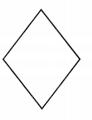(A) 3
(B) 5
(C) 4